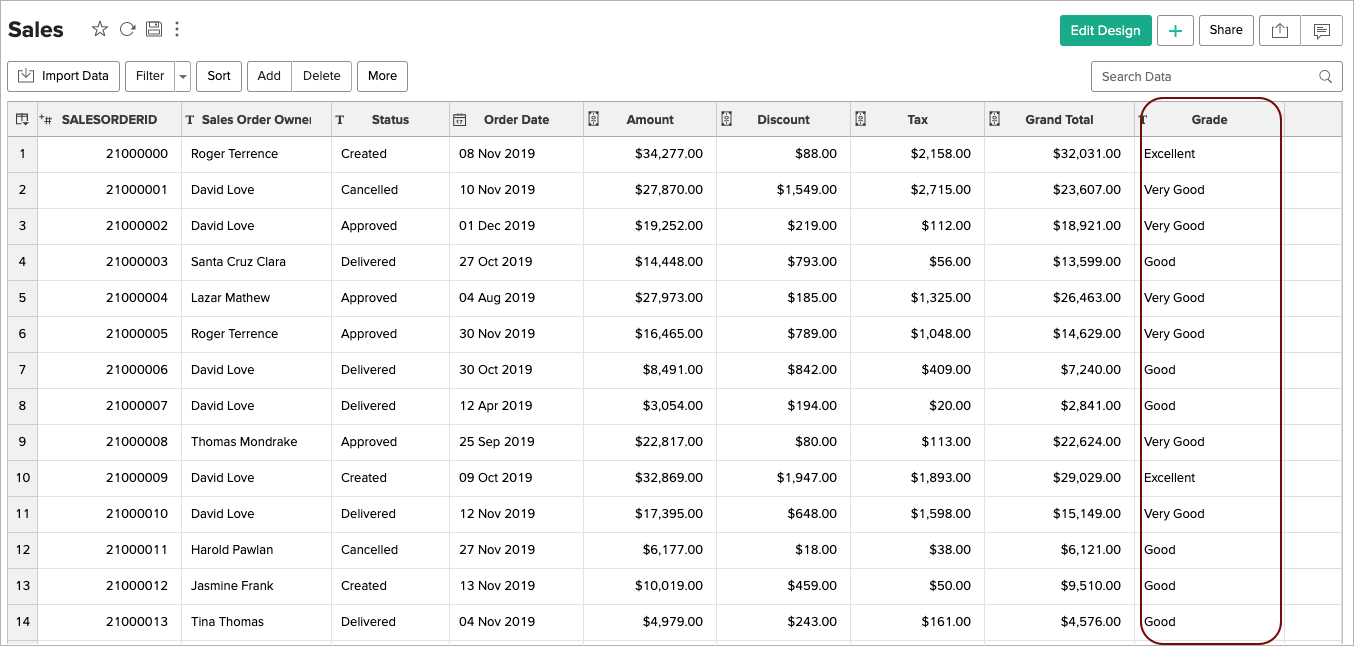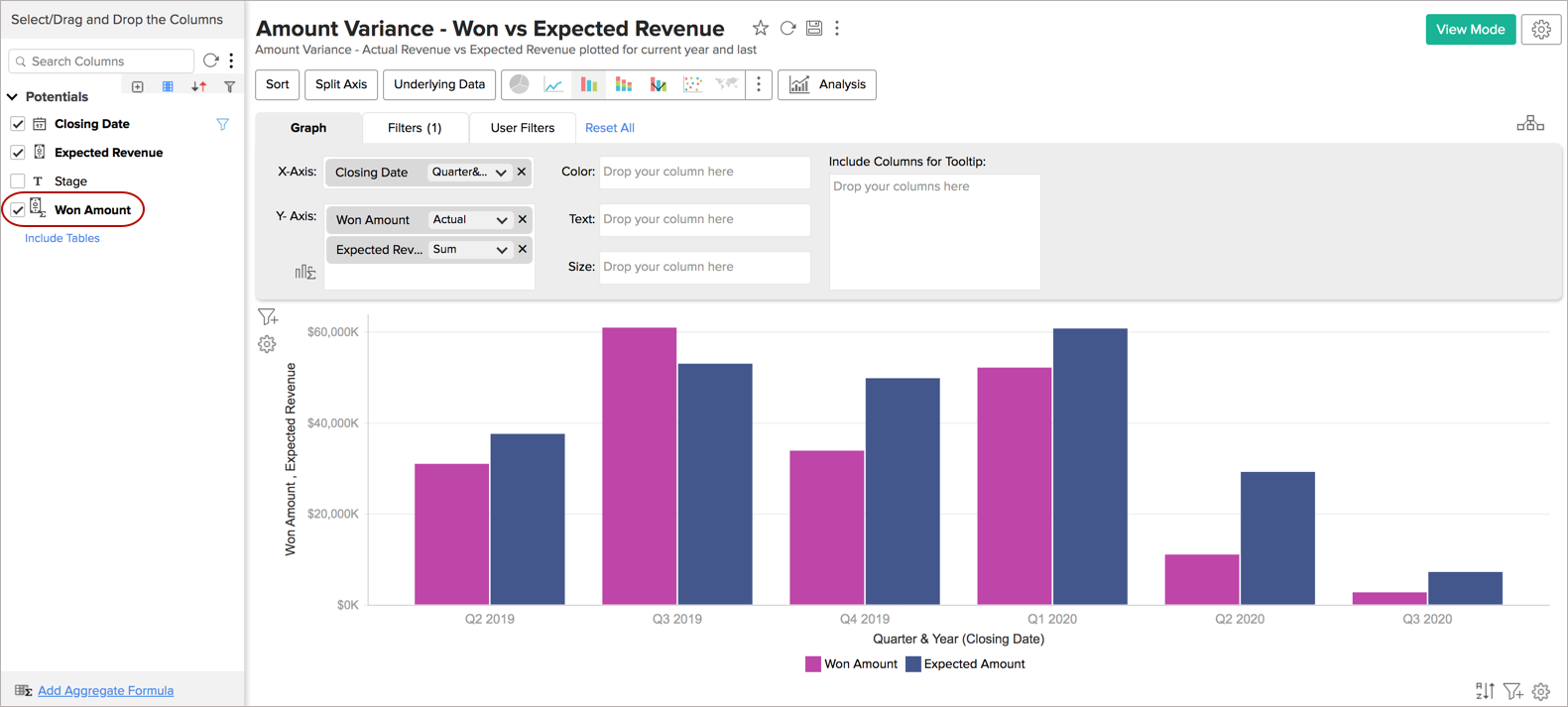# Creating Your Own Metrics with Formulas

Zoho Analytics with a powerful formula engine allows you to derive new business metrics from your data. This allows you to perform complex analysis and get the required insights from your data set.

Zoho Analytics provides a set of in-built functions for well-known specific calculations. You can simply apply these functions over your data or create your own formula expression with these functions for complex computations.

There are 3 types of formulas that you can use to define your metrics:

## Formula Column

Formula column allows you to create a new computed column in your data table based on a formula expression that you provide.

Zoho Analytics provides a wide range of Logical, Statistical, Date and String functions. You can combine these functions along with arithmetic operators like +, -, / and *  to formulate the formula column.  The result of the calculation will be saved as a new column in your data table.

Example: ‌The below is an example of Grading the sales based on sales amount using a Nested If formula. The gradings derived are Excellent, Very Good, Good, Poor, and Others.

if("Amount" >= 0 and "Amount" <= 500, 'Poor', if("Amount" >500 and "Amount" <= 15000, 'Good', if("Amount" > 15000 and "Amount" <= 30000, 'Very Good', if("Amount" > 30000, 'Excellent', 'Others'))))The defined formula columns can be used in any report creation similar to other columns in the data table. Click here to know more about Formula Columns.

## Aggregate Formula

Aggregate Formula allows you to create powerful metrics using formulas to address your business needs. These metrics often serve as the Key business performance indicators in your analysis & reporting.

The result of an Aggregate Formula is always a numeric value and will be computed for each data record/group in a report in which it is used. Unlike Formula Column, this will not be added as another column in the base table. They will just be associated with the table on which they have been created and can be used in creating reports (Charts, Pivot Tables, and Summary Views) like any other table column.

Example: The below example will calculate the Won Amount in sample CRM data. It sums the amount of all leads whose stage is "Closed Won"

sumif("Potentials"."Stage" = 'Closed Won',"Potentials"."Amount")